Chapter 6 Lines and Angles Class 9 NCERT CBSE Download in Pdf

(1) Point - We often represent a point by a fine dot made with a fine sharpened pencil on a piece of paper.

(2) Line - A line is completely known if we are given any two distinct points. Line AB is represented by as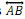. A line or a straight line extends indefinitely in both the directions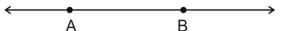(3) Line segment - A part (or portion) of a line with two end points is called a line segment.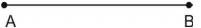(4) Ray - A part of line with one end point is called a ray.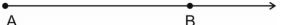(5) Collinear points - If three or more points lie on the same line, they are called collinear points otherwise they are called non-collinear points.

Types of Angles -
(1)Acute angle - An acute angle measure between 0o and 90o
(2) Right angle - A right angle is exactly equal to 90o.
(3)Obtuse angle - An angle greater than 90o but less than 180o
(4 )Straight angle - A straight angle is equal to 180o.
(5)Reflex angle - An angle which is greater than 180o but less than 360o is called a reflex angle.
(6)Complementary angles - Two angles whose sum is  90o are called complementary angles.
(7)Supplementary angle - Two angles whose sum iis 180 are called supplementary angles.
(8)Adjacent angles - Two angles are adjacent, if they have a common vertex, a common arm and their non common arms are on different sides of common arm.
(9)Linear pair - Two angles form a linear pair, if their non-common arms form a line.
(10)Vertically opposite angles - Vertically opposite angles are formed when two lines intersect each other at a point.

TRANSVERSAL

(a) Corresponding angles
(b) Alternate interior angles
(c) Alternate exterior angles
(d) Interior angles on the same side of the transversal.

•If a transversal intersects two parallel lines, then
(i) each pair of corresponding angles is equal.
(ii) each pair of alternate interior angles is equal.
(iii) each pair of interior  angle  on  the  same  side  of  the  transversal  is supplementary.

• If a transversal interacts two lines such that, either
(i) any one pair of corresponding angles is equal, or
(ii) any one pair of alternate interior angles is equal or
(iii) any one pair of interior angles on the same side of he transversal  is supplementary then the lines are parallel.

• Lines which are parallel to a given line are parallel to each other.

• The sum of the three angles of a triangle is 180o.

• If a side of a triangle is produced, the exterior angle so formed is equal to the sum of the two interior opposite angles.

### Section - A

Q.1 In the given figure,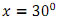The value of y is
(a)10o
(b)40o
(c) 36o
(d) 45o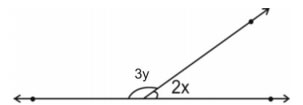Q.2 An exterior angle of a triangle is 750  and its two interior opposite angles are equal. Each of these equal angles is
(a) 105o
(b) 50.5o
(c) 52o
(d) 37.5o

Q.3 The compliment of an angle 'm' is:
(a) m
(b) 90o+m
(c) 90o-m
(d)m ×90o

Q.4If one angle of a triangle is equal to the sum of the other two equal angles, then the triangle is
(a) an isosceles triangle
(b)an obtuse triangle
(c)an equilateral triangle
(d) a right triangle

Q.5 In the given figure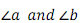form a linear pair if a-b = 100o then a and b are
(a)120o, 20o
(b) 40o, 140o
(c) 50o, 150o
(d) 140o, 40o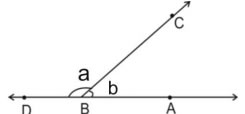Q.6 Angle of a triangle are in the ratio 2 : 4 : 3. The smallest angle of the triangle is

(a) 60o
(b) 40o
(c) 80o
(d) 20o

Section - B

Q.7 Two adjacent angles are equal. Is it necessary that each of these angles will be a right angle? Justify your answer.

Q.8 In the following figures which of the two lines are parallel and why?
(i)    (ii)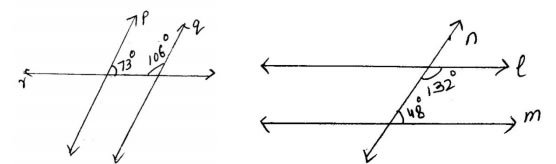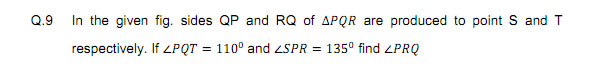Q.10  In the fig.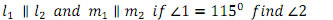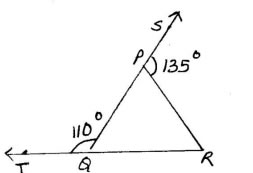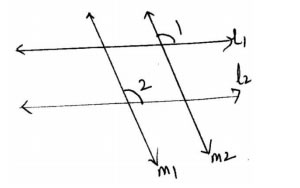Q.11 Sum of two angles of a triangle is 90o  and their difference is 50o. Find all the angles of the triangle.
Q.12  In the adjoining figure,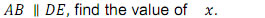.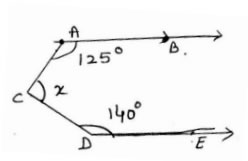Section - C

Q.13  In the given figure AB and CD intersect each other at O.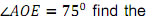If  find the value of x,y and z.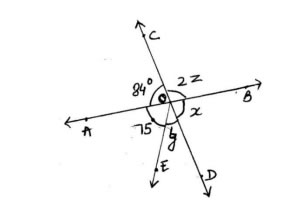Q.14  Prove that vertically opposite angle are equal.

Q.15  In the given figure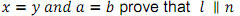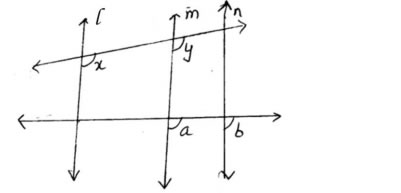Q.16  In the given figure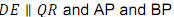are bisectors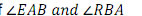respectively find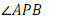Q.17  The angles of a triangle are in the ratio 2: 3: 5 find the angles of the triangle.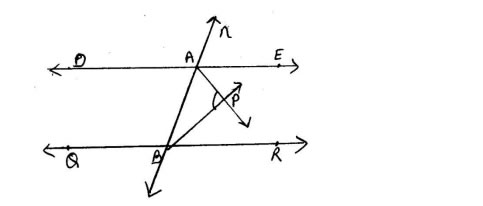Q.18  Find x and y in the following figure.

Q.19  In figure find x.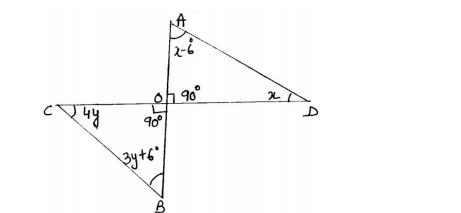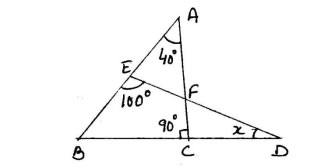Section - D

Q.20 Prove that sum of the angles of triangle is 180o.

Q.21 Prove that sum of the angles of a hexagon is 720o.

Q.22 The angles of a triangle are find the value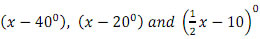of x.

Q.23 In the given figure, AD and CE are the angle bisectors of respectively If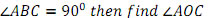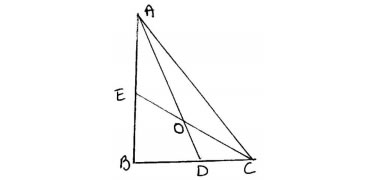Q.24  A transversal intersects two parallel lines. Prove that the bisectors of any pair of corresponding angle so formed are parallel.

(1) b
(2) d
(3) c
(4) a,d
( 5) d
(6) b
(9) 65o
(10) 115o
(11) 20o, 70o, 90o
(12) 95o
(13) 84o, 21o, 48o
(16)90o
(17) 36o, 54o, 90o
(18) 48o, 12o
(19) 30o
(22) 100o
(23) 135o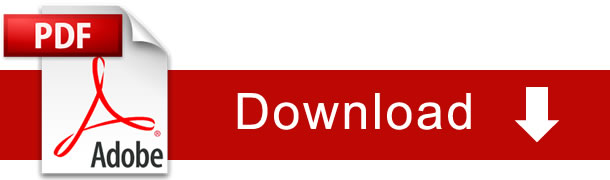### NCERT Books Free Pdf Download for Class 5, 6, 7, 8, 9, 10 , 11, 12 Hindi and English Medium

 Mathematics Biology Psychology Chemistry English Economics Sociology Hindi Business Studies Geography Science Political Science Statistics Physics Accountancy